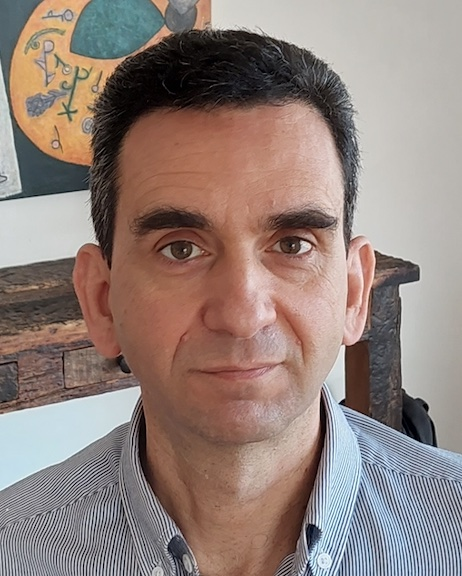Seminars

# Stavros Komineas (University of Crete) Dynamics of skyrmions and of topologically trivial solitons

Zoom onlyAbstract.
Skyrmionic textures are found as minimum energy solutions in magnetic materials where the chiral Dzyaloshinskii-Moriya interaction is present. They are characterized by an integer-valued topological invariant called the skyrmion number Q in this context. The model supports (i) an axially symmetric skyrmion with Q=1, (ii) an axially symmetric texture with Q=0 that is called the skyrmionium, (iii) bimeron configurations that are understood as transformations of the Q=1 skyrmion , and (iv) a chiral droplet that is a topologically trivial, Q=0, skyrmion-antiskyrmion configuration [2,3].

We will introduce the Landau-Lifshitz equation for the statics and dynamics of the magnetization in ferromagnets. We will present a fundamental relation that links topology and magnetization dynamics . A straightforward result is that topological textures (nonzero Q) are spontaneously pinned and follow Hall dynamics, while topologically trivial textures (Q=0) are accelerated by forces and follow Newtonian dynamics [4,5]. The laws are examplified by simulating the dynamics of the above-mentioned four skyrmionic textures [3,5].

It is gradually understood that skyrmionic textures are realizations of fractons, a type of unusual particle of restricted mobility discussed within spin lattice models and higher-rank gauge theories .

References.

 A. O. Leonov and I. Kezsmarki, Phys. Rev. B 96, 014423 (2017); D. Bachmann, M. Lianeris, S. Komineas, Phys. Rev. B (2023).
 V. M. Kuchkin et al, Phys. Rev. B 102, 144422 (2020)
 N. Sisodia, P.K. Muduli, K. Papanicolaou, S. Komineas, Phys. Rev. B 103, 024432 (2021).
 N. Papanicolaou, T.N. Tomaras, Nucl. Phys. B 360, 425 (1991).
 S. Komineas, N. Papanicolaou, Phys. Rev. B 92, 064412 (2015).
 M. Pretko, X. Chen, Y. You, Int. J. Mod. Phys. A 35, 2030003 (2021); Y.-H. Du, U. Mehta, D.X. Nguyen, and D.T. Son, SciPost Phys. 12, 050 (2022).

Back to List

pagetop